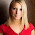## Saturday, June 21, 2014

### 9999=4 8888=8 1919=? Numerical Counting Puzzle

Now there is a puzzle, which will shake you:
9999=4
8888=8
1816=3
1212=0
1919=?
Solve the puzzle.

Solution:
The puzzle needs you to calculate the number of circles in the numbers.

For instance - 9 has one circle in it. And then 8 has 2

Hence see as below:
9999=4 (each 9 has 1 circle)
8888=8 (each 8 has 2 circles so 2 x 4 = 8)
1816=3 (8 has one circle and 6 has 1 so 2)
1212=0 (no circles in 1 & 2)
Hence, 1919=2 ( 2 circles of the number 9)

I have also seen another solution. THough not a clean one, but still the logic doesnot smells so foul:
See, sum of 9999 is equal to 4. Therefore, each 9 is equivalent to 1.
See 1212 is equal to 0. So 1 and 2 are equivalent to 0.
Therefore, 1919 is (0+1+0+1), so it is equivalent to 2.

--Enjoy

1.You say circles. Why? Doesn't make sense.

1.Yes....the solution to this is different
The above given solution is wrong
Here is the link to the correct solution

2.I appreciate several from the Information which has been composed, and especially the remarks posted I will visit once more.  Kreuzworträtsel Hilfe

3.Incredibly composed blog that has transfixed its group of onlookers.
Resumeyard

4.Add the equivalents. 9=1 8=2 7=3 6=4 5=5 4=7 1/2 3=4 1/2 2=1 1/2 1=-1 1/2 Jeff Lesmeister

5.Other logic can be look at the number of factors each has and give values according to that. 9 has 2 factors , 8 has 3 factors 2 has only one factor , so for one value zero , 2 value one , 3 value 2 and so on . Later add values.